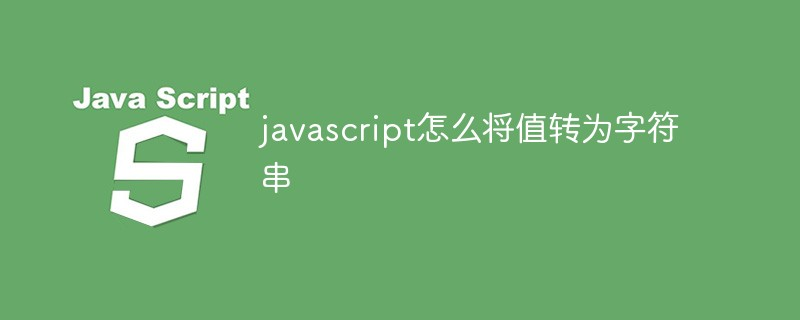# javascript怎么将值转为字符串JavaScript中将值转换为字符串的五种方法

```const value = 12345;
// Concat Empty String
value + '';
// Template Strings
`\${value}`;
// JSON.stringify
JSON.stringify(value);
// toString()
value.toString();
// String()
String(value);
// RESULT
// '12345'```

```const string = "hello";
const number = 123;
const boolean = true;
const array = [1, "2", 3];
const object = {one: 1 };
const symbolValue = Symbol('123');
const undefinedValue = undefined;
const nullValue = null;```

```string + ''; // 'hello'
number + ''; // '123'
boolean + ''; // 'true'
array + ''; // '1,2,3'
object + ''; // '[object Object]'
undefinedValue + ''; // 'undefined'
nullValue + ''; // 'null'
// ⚠️
symbolValue + ''; // ❌ TypeError```

````\${string}`; // 'hello'
`\${number}`; // '123'
`\${boolean}`; // 'true'
`\${array}`; // '1,2,3'
`\${object}`; // '[object Object]'
`\${undefinedValue}`; // 'undefined'
`\${nullValue}`; // 'null'
// ⚠️
`\${symbolValue}`; // ❌ TypeError```

JSON.stringify（）

```JSON.stringify(string); // '"hello"'
JSON.stringify(number); // '123'
JSON.stringify(boolean); // 'true'
JSON.stringify(array); // '[1,"2",3]'
JSON.stringify(object); // '{"one":1}'
JSON.stringify(nullValue); // 'null'
JSON.stringify(symbolValue); // undefined
JSON.stringify(undefinedValue); // undefined```

.toString（）

```string.toString(); // 'hello'
number.toString(); // '123'
boolean.toString(); // 'true'
array.toString(); // '1,2,3'
object.toString(); // '[object Object]'
symbolValue.toString(); // 'Symbol(123)'
// ⚠️
undefinedValue.toString(); // ❌ TypeError
nullValue.toString(); // ❌ TypeError```

String（）

```String(string); // 'hello'
String(number); // '123'
String(boolean); // 'true'
String(array); // '1,2,3'
String(object); // '[object Object]'
String(symbolValue); // 'Symbol(123)'
String(undefinedValue); // 'undefined'
String(nullValue); // 'null'```

【推荐学习：javascript高级教程• 相关标签：javascript 字符串
• 相关文章

相关视频